1. /
2. CBSE
3. /
4. Class 07
5. /
6. Mathematics
7. /
8. NCERT Solutions for Class...

# NCERT Solutions for Class 7 Maths Exercise 15.2### myCBSEguide App

Download the app to get CBSE Sample Papers 2023-24, NCERT Solutions (Revised), Most Important Questions, Previous Year Question Bank, Mock Tests, and Detailed Notes.

NCERT solutions for Maths Visualizing Solid Shapes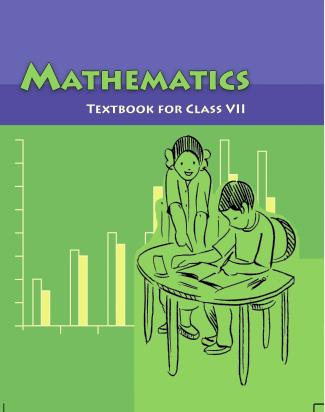## NCERT Solutions for Class 7 Maths Visualizing Solid Shapes

###### Questions 1. Use isometric dot paper and make an isometric sketch for each one of the given shapes: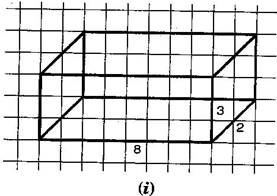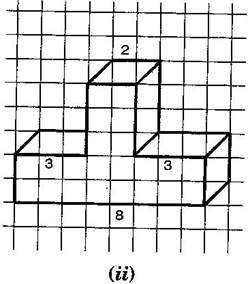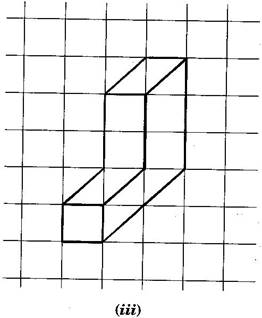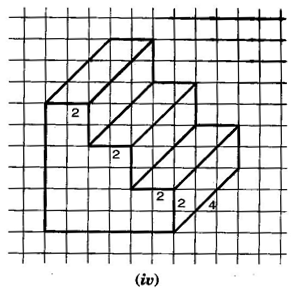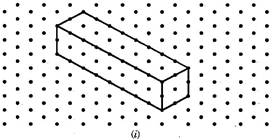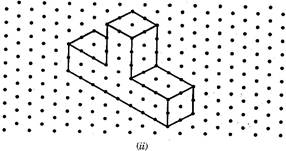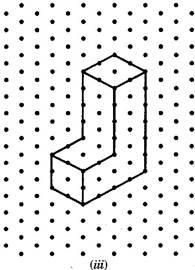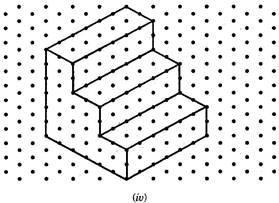NCERT Solutions for Class 7 Maths Exercise 15.2

###### Questions 2. The dimensions of a cuboid are 5 cm, 3 cm and 2 cm. Draw three different isometric sketches of this cuboid.

Answers : The dimensions of given cuboid are 5 cm, 3 cm and 2 cm: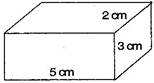Three different isometric sketches are: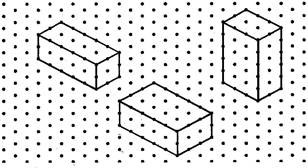NCERT Solutions for Class 7 Maths Exercise 15.2

###### Questions 3. Three cubes each with 2 cm edge are placed side by side to form a cuboid. Sketch an oblique or isometric sketch of this cuboid.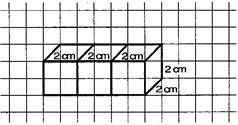Isometric sketch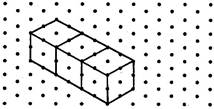NCERT Solutions for Class 7 Maths Exercise 15.2

###### Questions 4. Make an oblique sketch for each one of the given isometric shapes: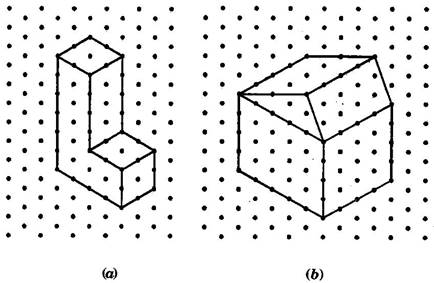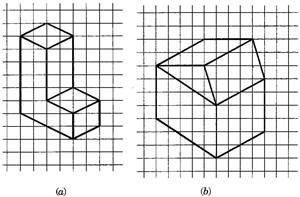NCERT Solutions for Class 7 Maths Exercise 15.2

###### Questions 5. Give (i) an oblique sketch and (ii) an isometric sketch for each of the following:

(a) A cuboid of dimensions 5 cm, 3 cm and 2 cm. (Is your sketch unique?)

(b) A cube with an edge 4 cm long.

Answers : (a) A cuboid of dimension 5 cm, 3 cm and 2 cm.

(i) Oblique sketch (ii) Isometric sketch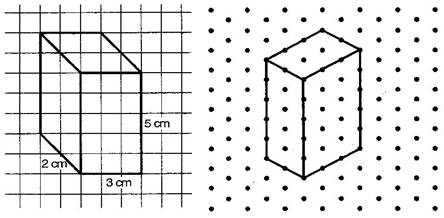(b) A cube with an edge 4 cm long.

(i) Oblique sketch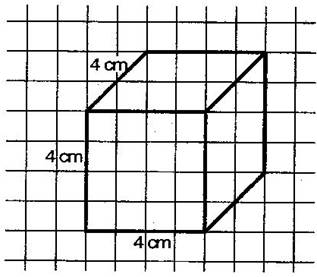(ii) Isometric sketch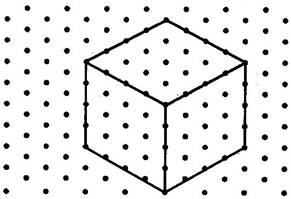NCERT Solutions for Class 7 Maths Exercise 15.2

###### Questions 6. An isometric sheet is attached at the end of the book. You could try to make on it some cubes or cuboids of dimensions specified by your friend.

Answers : Cubes and cuboids shapes on isometric sheet given below: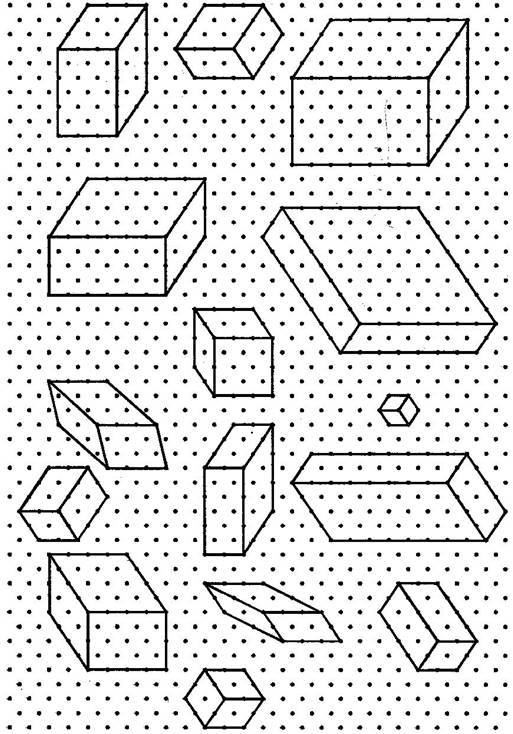You can also draw more shapes of cubes and cuboids.

## NCERT Solutions for Class 7 Maths Exercise 15.2

NCERT Solutions Class 7 Maths PDF (Download) Free from myCBSEguide app and myCBSEguide website. Ncert solution class 7 Maths includes text book solutions from Class 7 Maths Book . NCERT Solutions for CBSE Class 7 Maths have total 15 chapters. 7 Maths NCERT Solutions in PDF for free Download on our website. Ncert Maths class 7 solutions PDF and Maths ncert class 7 PDF solutions with latest modifications and as per the latest CBSE syllabus are only available in myCBSEguide.

## CBSE app for Students

To download NCERT Solutions for Class 7 Maths, Social Science Computer Science, Home Science, Hindi English, Maths Science do check myCBSEguide app or website. myCBSEguide provides sample papers with solution, test papers for chapter-wise practice, NCERT solutions, NCERT Exemplar solutions, quick revision notes for ready reference, CBSE guess papers and CBSE important question papers. Sample Paper all are made available through the best app for CBSE students and myCBSEguide website.Test Generator

Create question paper PDF and online tests with your own name & logo in minutes.myCBSEguide

Question Bank, Mock Tests, Exam Papers, NCERT Solutions, Sample Papers, Notes

### 5 thoughts on “NCERT Solutions for Class 7 Maths Exercise 15.2”

1. Thanks

2. Good job

3. Thank you

4. Ok thank God I don’t do

5. Good answers easy method ??